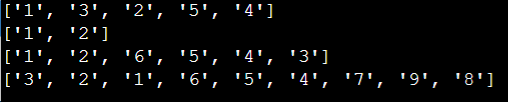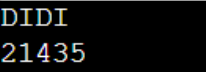New update is available. Click here to update.

# Form Minimum Number from Given Sequence

## Introduction

The stated problem "Form minimum number from given sequence" had been asked in Amazon Entrance Examination. Alongside it is one of those problems that checks an individual's critical thinking by analyzing the most fundamental approach and the basic simple mathematics of the programmer.

We will discuss how to form a minimum number from a given sequence. Let's check the problem statement first to know more about the problem.

### Problem Statement

Given problem "Form minimum number from given sequence" states that we have a pattern containing only I's and D's in it, where I means increasing and D means decreasing. We have to write a schematic code for the above-mentioned problem. One rule is that you take digits from 1 to 9 and don't repeat any digits.

### Sample Examples

Example 1

Input

``DIDI``

Output

``21435``

Explanation: The pattern of the elementary problem is DIDI, and the output number is 21435. There is a decrement in number for the first letter, "D," i.e., 2 to 1. There is an increment in number for the second letter, "I," i.e., from 1 to 4, similarly to other letters.

Example 2

Input

``DDIDDIID``

Output

``321654798``

Explanation: The pattern of the elementary problem is DDIDDIID, and the output number is 321654798. There is a decrement in number for the first letter, "D," i.e., 3 to 2. There is an increment in number for the third letter, "I," i.e., from 1 to 6, similarly to other letters.

## Brute Force Approach

In the Brute Force Approach, we assume the smallest number as the solution and continue shifting the digits until we reach a D. There's no need to go back to get the index. Follow the steps given below to understand the given problem more precisely:

2. Iterate to the end of the digits list, keeping track of the first D in a sequence of Ds, starting with the second digit (index 1) and first character (D)
• When we come across a D:
Change the current index digit to the first D in the sequence.

• When we come across an I:
Reset the last known location of D. There's nothing to move because the digit is correctly placed.

### Implementation

``````def sequence(s: str):

if not s or len(s) <= 0:
return ""
main_list = ["1"]
for i in range(1, len(s) + 1):
main_list.append(f'{i + 1}')

last_D = -1
for i in range(1, len(main_list)):
if s[i - 1] == 'D':
if last_D < 0:
last_D = i - 1
v =main_list[i]
del main_list[i]
main_list.insert(last_D, v)
else:
last_D = -1

return main_list

# Function call
print(sequence("IDID"))
print(sequence("I"))
print(sequence("IIDDD"))
print(sequence("DDIDDIID" ))``````

#### Output#### Time Complexity

The time complexity of the stated problem “Form minimum number from given sequence” is O(N), because the loop will run N times in the stated problem. Here, N is the number of characters in the given sequence.

#### Space Complexity

The space complexity of the stated problem “Form minimum number from given sequence” is O(N), because it is taking N times space in the given solution. Here, N is the number of characters in the given sequence.

## Optimized Approach

1. In the Optimized Approach, the first step is to traverse the given array char by char till the end of the array.

2. If the value at the current location is 'I':
• Now calculate the count of consecutive D's after the present element 'I' and store it in a variable where the number of D's are stored.

• If it is the first element, make the maximum variable equal to the number of consecutive D's present plus 2 to print the first two elements in the output.

• Suppose if it is not the first element, then do the maximum is equal to the previous maximum plus the number of D's plus 1 and make the lastPrinted equal to the maximum and then print last_printed.

3. And, if the value at the current location is 'D':
• If D is the first element, then calculate the number of consecutive D after the present element 'D' and store it in a variable where the number of D's are stored.

• Calculate the first digit to print based on no of D's. So, the maximum variable is equal to the number of consecutive D's present plus 2.

• If it is not the first element, decrement the lastPrinted and then print the lastPrinted.

### Implementation

``````#include<bits/stdc++.h>
using namespace std;
void LeastPrint(string str)
{
int start = 1, pos = 0;
vector<int>v;
if(str=='I')
{
v.push_back(1);
v.push_back(2);
start = 3;
pos = 1;
}
else
{
v.push_back(2);
v.push_back(1);
start = 3;
pos = 0;
}
for (int i=1; i<str.length(); i++)
{
if (str[i]=='I')
{
v.push_back(start);
start++;
pos = i+1;
}
else
{
v.push_back(v[i]);
for (int j=pos; j<=i; j++)
v[j]++;
start++;
}
}
for(auto u: v)
{
cout<<u;
}
cout<<endl;
}
int main()
{
string str;
cin>>str;
LeastPrint(str);
return 0;
}``````

Input

``DIDI``

#### Output#### Time Complexity

The time complexity of the stated problem “Form minimum number from given sequence” is O(n), because the loop will run n times in the stated problem. Here, N is the number of characters in the given sequence.

#### Space Complexity

The space complexity of the stated problem “Form minimum number from given sequence” is O(1) as we did not use any extra data structure for any operation.

### What are the other approaches to solve the “Form minimum number from a given sequence” problem?

There are many numbers of approaches to solving this elementary problem. Some of them are mentioned below:

• Using two pointers

### What is Linear data structure?

Linear Data Structure is a collection of data values having the same data types and is stored in a contiguous memory location. An array of elements is an example of a Linear Data Structure.

### What do you mean by Doubly Linked List in data structure?

Doubly Linked List in the data structure is a type of Linked List where movement is possible in both ways that are either forward or backward easily as compared to normal Linked List or SIngle Linked List.

## Conclusion

We have extensively discussed how to form a minimum number from the given sequence in this article. We started by introducing the topic, problem statement, example, pseudocode, and implementation, and finally concluded with the time complexity of the algorithm.

We hope that this blog has helped you enhance your knowledge regarding how to form a minimum number from a given sequence and if you would like to learn more, check out our other articles on the problems like the Largest sum of averagesDiamond TreeKth Distinct String in an Array and many more on our platform CodeStudio.

For peeps out there who want to learn more about Data Structures, Algorithms, Power programming, JavaScript, or any other upskilling, please refer to guided paths on CodeStudio. Enroll in our courses, go for mock tests, solve problems available, and interview puzzles. Also, you can put your attention towards interview stuff- interview experiences and an interview bundle for placement preparations.

Do upvote our blog to help other ninjas grow.